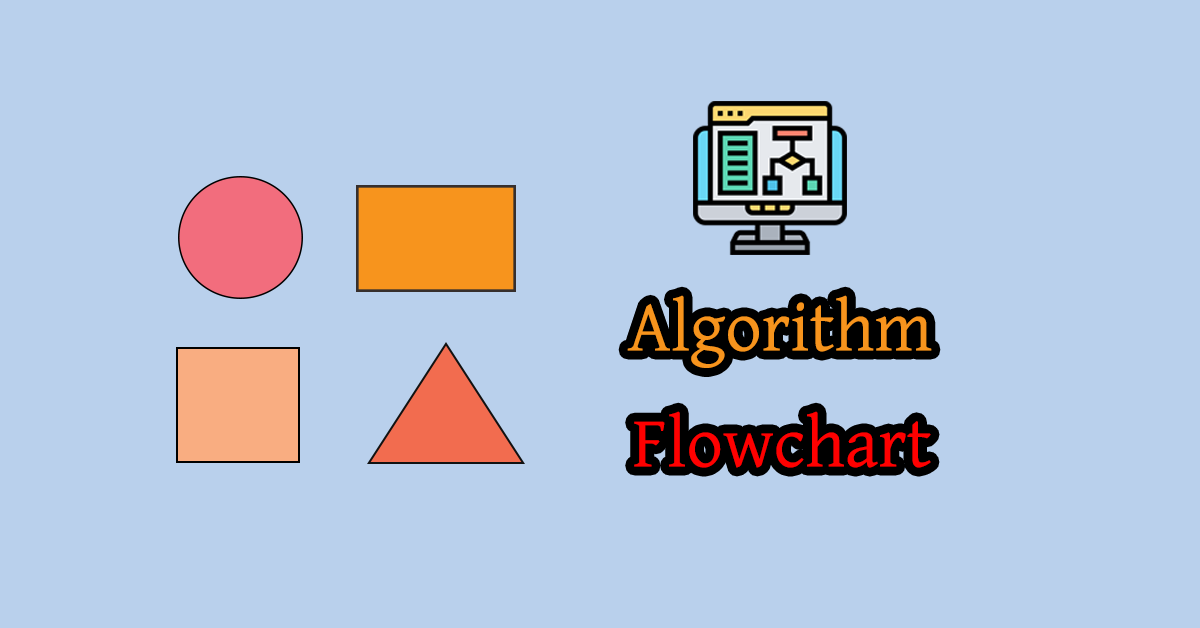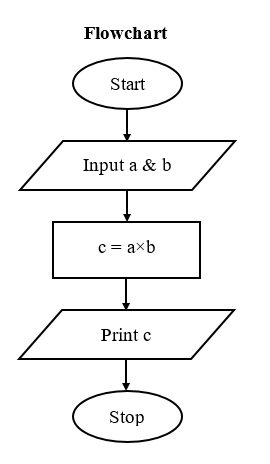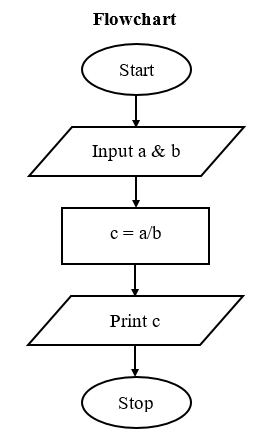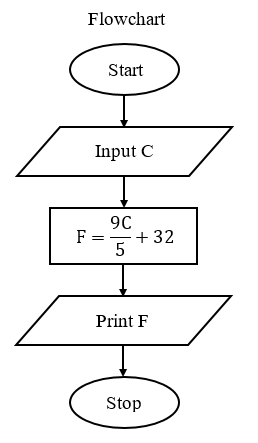# Fifth Chapter Lesson-5: Basic Mathematical problem related algorithm & flowchart.## At the end of this lesson-

1. You will be able to write algorithm & flowchart for adding two numbers.

2. You will be able to write algorithm & flowchart for subtracting two numbers.

3. You will be able to write algorithm & flowchart for multiplying two numbers.

4. You will be able to write algorithm & flowchart for dividing two numbers.

5. You will be able to write algorithm & flowchart for converting temperature from Celsius to Fahrenheit.

6. You will be able to write algorithm & flowchart for converting temperature from Fahrenheit to Celsius.

7. You will be able to write algorithm & flowchart for determining area of a triangle where base and height have been given.

8. You will be able to write algorithm & flowchart for determining area of a triangle where three arms have been given.

9. You will be able to write algorithm & flowchart for determining area of a rectangle.

10. You will be able to write algorithm & flowchart for determining area of a circle.

## Go for Bangla Version

1. Algorithm & flowchart for adding two numbers.

Algorithm

Step-1: Start.

Step-2: Input two numbers in variable a & b.

Step-3: Calculate c = a+b.

Step-4: Print value of c.

Step-5: Stop.Practice: Write an algorithm & flowchart for adding five integer numbers.

2. Algorithm & flowchart for subtracting two numbers.

Algorithm

Step-1: Start.

Step-2: Input two numbers in variable a & b.

Step-3: Calculate c = a-b.

Step-4: Print value of c.

Step-5: Stop.3. Algorithm & flowchart for multiplying two numbers.

Algorithm

Step-1: Start.

Step-2: Input two numbers in variable a & b.

Step-3: Calculate c = a×b.

Step-4: Print value of c.

Step-5: Stop.Practice: Write an algorithm & flowchart for multiplying five integer numbers.

4. Algorithm & flowchart for dividing two numbers.

Algorithm

Step-1: Start

Step-2: Input two numbers in variable a & b.

Step-3: Calculate c = a/b.

Step-4: Print value of c.

Step-5: Stop.Practice: Write an algorithm & flowchart for determining average of five integer numbers.

5. Algorithm & flowchart for converting temperature from Celsius to Fahrenheit.

Relation among different temperature scale C/5 = F-32 / 9 = K-273 / 5

Algorithm

Step-1: Start.

Step-2: Input Celsius temperature in variable C.

Step-3: Calculate F=(9C/5)+32.

Step-4: Print value of F.

Step-5: Stop.Practice: Write an Algorithm & flowchart for converting temperature from Celsius to Kelvin.

6. Algorithm & flowchart for converting temperature from Fahrenheit to Celsius.

Relation among different temperature scale C/5 = F-32 / 9 = K-273 / 5

Algorithm

Step-1: Start.

Step-2: Input Fahrenheit temperature in variable F.

Step-3: Calculate C=((F-32)5)/9.

Step-4: Print value of C.

Step-5: Stop.Practice: Write an Algorithm & flowchart for converting temperature from Fahrenheit to Kelvin.

7. Algorithm & flowchart for determining area of a triangle where base and height have been given.

Theory for determining the area of a triangle where base and height of triangle have been given,  Area=1/2×base×height

Algorithm

Step-1: Start.

Step-2: Input base and height of triangle in variable b & h.

Step-3: Calculate  area = 1/2×b×h.

Step-4:  Print value of area.

Step-5: Stop.8. Algorithm & flowchart for determining area of a triangle where three arms have been given.

Theory for determining the area of a triangle where three arms of triangle are a, b and c,                           Area= √(s(s-a)(s-b)(s-c))   [ s= half of perimeter]

half of perimeter, s=(a+b+c)/2

Algorithm

Step-1: Start.

Step-2: Input three arms of a triangle in variable a, b and c.

Step-3: Calculate s = (a+b+c)/2.

Step-4: Calculate area = √(s(s-a)(s-b)(s-c)).

Step-5: Print value of area.

Step-6: Stop.

Flowchart:9. Algorithm & flowchart for determining area of a rectangle.

Theory for determining area of rectangle where length and width have been given, Area=length×width

Algorithm

Step-1: Start.

Step-2: Input length and width of rectangle in variable l and w.

Step-3: Calculate area = l×w.

Step-4: Print value of area.

Step-5: Stop.Practice: Write an Algorithm & flowchart for determining area of a parallelogram.

Practice: Write an Algorithm & flowchart for determining area of a square.

10. Algorithm & flowchart for determining area of a circle.

Theory for determining area of a circle where radius r, Area=πr2

Algorithm

Step-1: Start.

Step-2: Input radius of circle in variable r.

Step-3: Calculate area = πr2.

Step-4: Print value of area.

Step-5: Stop.## Lesson Evaluation-

Knowledge Based Questions:

Comprehension Based Questions:

Creative Questions:

Multiple Choice Questions:

Written by,

1.Daud hossain says: